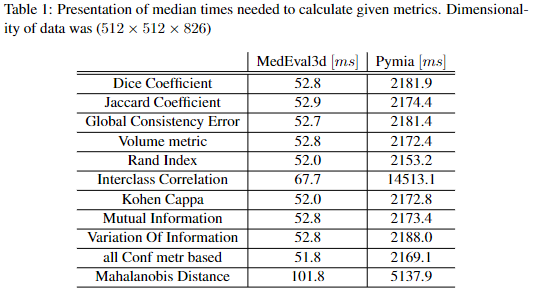## MedEval3D.jl

Set of CUDA accelerated medical segmentation metrics.
Author jakubMitura14
Popularity
1 Star
Updated Last
5 Months Ago
Started In
August 2021

# MedEval3D

Project with set of CUDA accelerated medical segmentation metrics.Mathemathical basis for metrics calculations are based on the work of the Taha et al. .

``````]add MedEval3D
``````

# Example

Example for calculating Mahalinobis distance

``````
using MedEval3D
using MedEval3D.BasicStructs
using MedEval3D.MainAbstractions

arrGold = CUDA.ones(3,3,3)
arrAlgoCPU = ones(3,3,3)
arrAlgoCPU[1,1,1]=0
arrAlgoCPU[3,3,3]=0
arrAlgoCPU[3,2,3]=0
arrAlgoCPU[3,2,2]=0
arrAlgo =CuArray(arrAlgoCPU)

conf= ConfigurtationStruct(md=true)
numberToLookFor = UInt8(1)

preparedDict=MainAbstractions.prepareMetrics(conf)

res= calcMetricGlobal(preparedDict,conf,arrGold,arrAlgo,numberToLookFor)
res.md # will give 0.127

``````

# Details

Programming model is based on the two phase metric evaluation.

First phase is invoked as a preparation step in order to calculate variables that are constant across kernel given image array dimensions. Those constants include thread block dimensions and number of required thread blocks to optimize occupancy using Occupancy API. Other constants are mainly related to precalculation of loop sizes and appropriate GPU memory allocations. Preparation step is designed to be invoked once for each dataset and can be cached and reused given image array size and GPU hardware will not change.

Second phase is invoked with an image array and gold standard segmentation together with variables calculated in the preparation step. Additionally to enable reliable calculation in multiple function invocations all data stractures are set to initial values (ussually 0).

For more theory You may look into paper that is currently in development https://www.overleaf.com/project/60f54dd02d12a4796b60026d. In case of any questions to this package or to the rest of medical segmentation framework (currently also visualization and segmentation tool at https://github.com/jakubMitura14/MedEye3d.jl) ask me on github or linkedIn https://www.linkedin.com/in/jakub-mitura-7b2013151/

For executing othe metrics we need to specify appropriate ConfigurtationStruct, we can do multiple metrics at once and it will run in similar time as single metric (most of the computations are reused)

First we need to specify input data

``````arrGold = CuArray(...) # 3 dimansional CUDA array representing gold standard mask
arrAlgo = CuArray(...) # 3 dimansional CUDA array representing output of our algorithm - we want to compare it against gold standard ...
``````

Next proper configuration

``````numberToLookFor = ... # number in arrGold and arrAlgo that is marking the structure of intrest for example 1
conf = ConfigurtationStruct(...) # we set in the Configuration struct to true field representing metric of intrest - we can mark multiple to true or just one - reference in the end of read me file.
``````

We invoke the preparation step - this needs to be invoked only once unless there is change in ConfigurtationStruct or in GPU hardware.

``````preparedDict=MainAbstractions.prepareMetrics(conf)
``````

Last step is invoking metrics

``````res = calcMetricGlobal(preparedDict,conf,arrGold,arrAlgo,numberToLookFor))
``````

Now we access the Result using the field name of the ResultMetrics struct (reference in the end)

Reference to ConfigurtationStruct and ResultMetrics

``````struct ConfigurtationStruct
dice::Bool = false #dice coefficient
jaccard::Bool = false #jaccard coefficient
gce::Bool = false #global consistency error
vol::Bool = false# Volume metric
randInd::Bool= false # Rand Index
ic::Bool= false # interclass correlation
kc::Bool= false # Kohen Cappa
mi::Bool= false # mutual information
vi::Bool= false # variation Of Information

mutable struct ResultMetrics
dice::Float64 = -1.0 #dice coefficient
jaccard::Float64 =  -1.0 #jaccard coefficient
gce::Float64 =  -1.0 #global consistency error
vol::Float64 =  -1.0 # Volume metric
randInd::Float64 = -1.0 # Rand Index
ic::Float64 = -1.0 # interclass correlation
kc::Float64 = -1.0 # Kohen Cappa
mi::Float64 = -1.0 # mutual information
vi::Float64 = -1.0 # variation Of Information
md::Float64 = -1.0 # mahalanobis distance
end

``````

# Benchmarks

All experiments were conducted on Windows PC (Intel Core I9 10th gen., GeforceRTX 3080), and Data from CT-ORG  dataset, on image of size (512×512×826). Time needed to calculate metrics was estimated in case of Julia code using BenchamrkTools.jl . For testing python libraries internal python module timeit was utilized. Results of experiments mentioned above are summarized in Figure 1, and in Table 1. In all cases data was already in RAM memory for CPU computation or in GPU memory for CUDA computations - hence memory transfer times were not included. As visible in the implementation of CUDA acceleration of described package in most cases led to from 40 up to 214 times shorter execution times. The only exception is in case of CUDA accelerated Monai Dice metric algorithm MedEval3D is slower (24.1 ms vs 52.8 ms) . However from all of the metrics described Monai implements only Dice metric, although admittedly most of others could be potentially calculated from Monai confusion matrix. What is also worth pointing out is a field in a table named ”all Conf metr based” this tested calculating all of the metrics jointly apart from interclass correlation and mahalanobis distance - time of execution in such case both in case of MedEval3d and Pymia algorithms was similar to calculation of just one of those metrics. This can be explained by the fact that in all of those cases the most computation intensive work is related to calculation of confusion matrix, and this calculation is reused in both packages between diffrent metrics. 11Figure 1: Comparison of median times needed to calculate given metrics in log scale, for Monai only CUDA accelerated algorithm was taken into account. Dimensionality of data was (512x512x826)If You will find usefull my work please cite it

``````@Article{Mitura2021,
author   = {Mitura, Jakub and Chrapko, Beata E.},
journal  = {Zeszyty Naukowe WWSI},
title    = {{3D Medical Segmentation Visualization in Julia with MedEye3d}},
year     = {2021},
number   = {25},
pages    = {57--67},
volume   = {15},
doi      = {10.26348/znwwsi.25.57},
keywords = {OpenGl, Computer Tomagraphy, PET/CT, medical image annotation, medical image visualization},
}

``````

 Taha, A.A., Hanbury, A. Metrics for evaluating 3D medical image segmentation: analysis, selection, and tool. BMC Med Imaging 15, 29 (2015). https://doi.org/10.1186/s12880-015-0068-x